China in Transition

# How is the Midpoint Rate of the Yuan against the U.S. Dollar Determined?"Closing rate on the preceding day + currency basket-based adjustment" rule starting to take root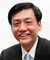Chi Hung KWAN
Consulting Fellow, RIETI

Since 2005, China has been seeking to move to a floating exchange rate system from the traditional one that focused on the stability of the yuan-to-dollar exchange rate (a de facto dollar peg system). In this process, the permissible range of the daily fluctuation (band) around the midpoint rate of the yuan to the dollar set by the authorities (hereinafter the "midpoint rate") has been widened step by step from +/- 0.3% to +/- 2%. In addition, when setting the midpoint rate, the closing rate on the preceding (trading) day that reflects the balance between supply and demand in the market and the "currency basket-based adjustment" that takes into account fluctuations in the exchange rates of major currencies against the dollar, have come to be emphasized. In this article, I will analyze how the midpoint rate is determined, focusing primarily on the "closing rate on the preceding day + currency basket-based adjustment" rule that is taking root.

## 1. The "closing rate on the preceding day + currency basket-based adjustment" rule

In recent years, the People's Bank of China (PBOC) has been working to develop a mechanism for formulating the midpoint rate of the yuan (the benchmark value). On August 11, 2015, it guided the yuan weaker by lowering the midpoint rate to reflect the prevailing market rate, while emphasizing the use of the closing rate on the preceding day as a reference when deciding the midpoint rate. In addition, on December 11, 2015, the China Foreign Exchange Trade System (CFETS) introduced the CFETS RMB Index, which shows the value of the yuan against a currency basket consisting of the currencies of 13 countries and regions. Using this index as a guide, a mechanism for formulating the midpoint rate of the yuan called the "closing rate on the preceding day + currency basket-based adjustment" rule has been established. (The second component by itself will be referred to as the "currency basket-based rule".) The PBOC explains the details of this rule as follows ("China Monetary Policy Report" first quarter of 2016, May 6, 2016).

The midpoint rate, which is announced every morning by the authorities, is calculated based on the quotations submitted by the market makers (financial institutions designated by the PBOC and the CFETS as banks that determine the interbank rate of the yuan). When estimating the midpoint rate, the market makers have to consider both the "closing rate on the preceding day" and the "currency basket-based adjustment." The "closing rate on the preceding day" is the closing rate of the yuan against the U.S. dollar in the interbank market as of 4:30 p.m. on the preceding day, which is supposed to reflect the supply-demand situation in the foreign exchange market. The "currency basket-based adjustment" is an adjustment made to the yuan-to-dollar exchange rate that is needed to keep the value of the yuan against the currency basket (e.g., the CFETS RMB Index) at the level of the preceding day amid fluctuations in the currencies that constitute the basket.

Every day before the interbank market opens, the market makers calculate the adjustment to the yuan-to-dollar exchange rate necessary to maintain the stability of the yuan against some currency baskets, taking into consideration of the fluctuations in the currencies composing those baskets against the dollar from the preceding day to the current day, and estimate the midpoint rate on the current day by adding the adjustment calculated onto the closing rate for the preceding day. Because the market makers make their own judgments by referring to three currency baskets—namely, the CFETS RMB Index, the effective yuan exchange rate of the Bank for International Settlement (BIS), and the special drawing rights (SDR) of the International Monetary Fund (IMF), their estimates vary. After excluding the highest and the lowest values from the quotations submitted by the market makers, the CFETS calculates the average value and announces it as the midpoint rate on the current day at 9:15 a.m.

The following numerical example helps to illustrate how the midpoint rate is determined under this rule. If the yuan-to-dollar exchange rate needs to be revalued by 100 basis points (bp, 1bp = 0.0001 yuan) to maintain the currency basket rate (e.g., the CFETS RMB Index) on the current day at the level of the preceding day in the event that the benchmark value of the midpoint rate of the yuan to the dollar on the preceding day is 6.5000 yuan and the closing rate on the preceding rate is 6.4950 yuan, the midpoint rate will be set at 6.4850 yuan, which is 150bp higher than the midpoint rate of the preceding day. Of the 150bp, 50bp reflect the change in the balance between demand and supply in the market, while 100bp reflect the "currency basket-based adjustment" (Figure 1).

Figure 1: Determining the Midpoint Rate Based on the "Closing Rate on the Preceding Day + Currency Basket-based Adjustment" Rule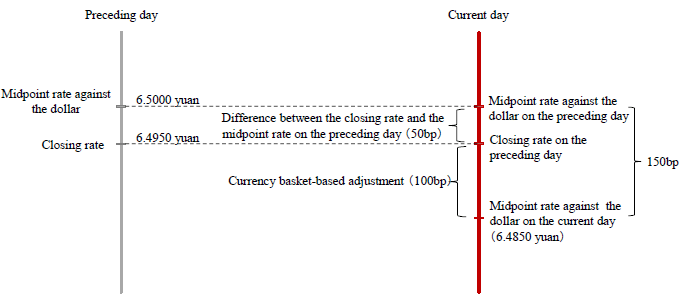Note: The market rate is not allowed to exceed the band of +/-2% around the midpoint rate on the current day.
Source: Compiled based on the "China Monetary Policy Report" for the first quarter of 2016 released by the PBOC on May 6, 2016.

Table 1: Composition of Three Currency Baskets Used for Reference by the Chinese Authorities

(Unit: %)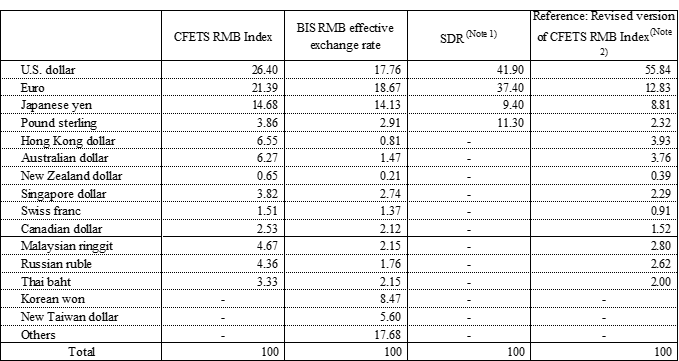Note 1: Although the Chinese yuan is set to join the constituent currencies of the SDR in October 2016, the values here show the weights that reflect the previous adjustment (in 2010).
Note 2: Our analysis suggests that the composition of the currency basket actually used by the authorities as a reference comes close to our revised version of the CFETS RMB Index.
Source: Compiled based on data of the CFETS, the BIS, and the IMF.
Figure 2: Basket Peg System vs. Dollar Peg System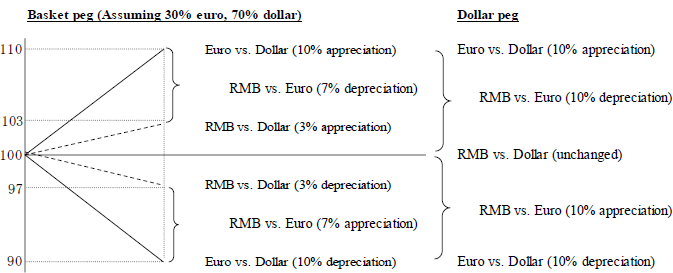Source: Compiled by the author.

Suppose, for example, that the respective weights of the euro and the dollar in a currency basket, which is used as the basis for deciding the yuan-to-dollar exchange rate, are 30% and 70%. In this case, if the euro appreciates against the dollar by 10%, the authorities make an adjustment so that the yuan appreciates by 3% against the dollar (10% × 30%), consequently causing the yuan to depreciate by 7% against the euro. In this manner, the yuan is linked with the euro at the rate of 30% against the dollar. Although the yuan-to-dollar exchange rate becomes more unstable (yuan appreciates by 3% rather than remaining the same) than in the dollar peg system, the yuan exchange rate against the euro becomes more stable (yuan depreciates by 7% rather than by 10%). In contrast, when the euro depreciates by 10% against the dollar, an adjustment is made by the authorities so that the yuan will depreciate by 3% against the dollar, consequently causing the yuan to appreciate by 7% against the euro (Note 1). Thus, even if the euro-to-dollar exchange rate fluctuates, the value of the yuan to the currency basket will remain unchanged (When the euro appreciates by 10%: -7% × 30% + 3% × 70% = 0; When the euro depreciates by 10%: 7% × 30% - 3% × 70% = 0) (Note 2).

## 3. How the "closing rate on the preceding day + currency basket-based adjustment" rule works in practice

To figure out how the "closing rate on the preceding day + currency basket-based adjustment" rule actually works in practice, I will analyze the determinants of the midpoint rate based on the two following hypotheses for the period after August 11, 2015, the date on which the yuan was devalued.

(Hypothesis I) The "closing rate on the preceding day" rule:
Midpoint rate on the current day = Closing rate on the preceding day ...Equation I

(Hypothesis II) The "closing rate on the preceding day + currency basket-based adjustment" rule:
Midpoint rate on the current day = Closing rate on the preceding day + Currency basket-based adjustment
⇒Midpoint rate on the current day - Closing rate on the preceding day = Currency basket-based adjustment ... Equation II

In the empirical analysis that follows, the left side of Equation I and Equation II corresponds to the actual value, and the right side corresponds to the theoretical value calculated based on these two hypotheses (simulation result). The difference between them (divergence of the actual value from the theoretical value) represents the error that cannot be explained by the hypotheses, and the smaller the error (in absolute term) is, the stronger is the explanatory power of the hypotheses (Figure 3 and Figure 4).

Figure 3: Verification of the "Closing Rate on the Preceding Day" Rule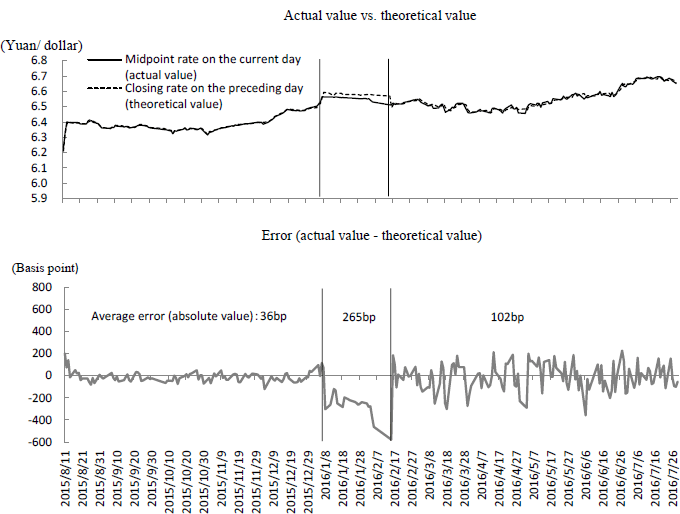Source: Compiled based on the CFETS (the midpoint rate on the current day) and Bloomberg (the closing rate on the preceding day).
Figure 4: Verification of the "Closing Rate on the Preceding Day + Currency Basket-based Adjustment" Rule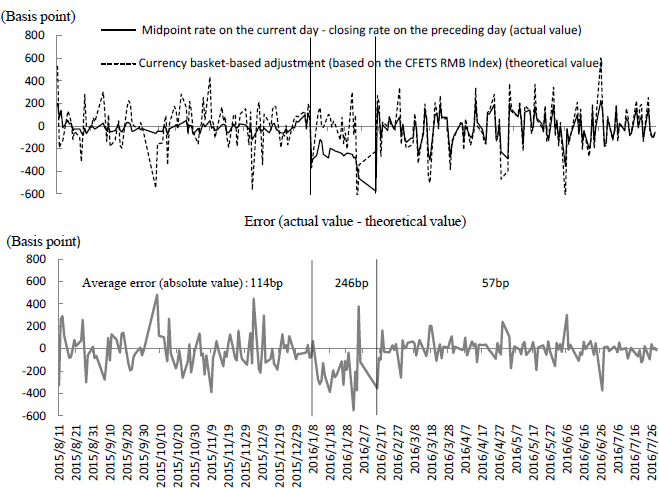Source: Compiled based on the CFETS (the midpoint rate on the current day, weights of the currency basket, exchange rates of currencies against the dollar) and Bloomberg (the closing rate on the preceding day).

To verify Hypothesis II, the theoretical value for the "currency basket-based adjustment" is calculated, following the explanation of the PBOC mentioned in Section 1, based on the CFETS RMB Index on which the authorities are believed to place the most importance (Note 3). In doing so, the exchange rates of the constituent currencies against the dollar and their changes from the preceding day (including changes in overseas markets after trading ended in the Chinese market) are calculated based on the midpoint rate of the yuan against each currency announced by the CFETS every morning (Note 4). While changes in the exchange rates were expressed by the rate of change (%) in the explanation in Section 2, they are converted into a basis point (bp) here to match the explanation of the authorities.

The period covered by this analysis can be divided into the following three sub-periods, judging from the changes in the size of errors over time in these two hypotheses.

In the first period from August 11, 2015 to January 7, 2016, the error (absolute value) is smaller and the explanatory power is stronger in the "closing rate on the preceding day" rule than in the "closing rate on the preceding day + currency basket-based adjustment" rule. This suggests that the closing rate on the preceding day served as a more useful reference for determining the midpoint rate, while the "currency basket-based adjustment" rule was not really taken into account.

Next, in the second period from January 8, 2016 to February 15, 2016, the error (absolute value) is large and the explanatory power as a hypothesis is low for both the "closing rate on the preceding day" rule and the "closing rate on the preceding day + currency basket-based adjustment" rule. In this period, the midpoint rate of the yuan was consistently set at a higher level than the closing rate on the preceding day (265bp higher on average). This may be interpreted as an emergency action taken by the authorities to lower growing expectations for the yuan to depreciate further.

In the third period from February 16, 2016 to July 29, 2016, the error (absolute value) is smaller and the explanatory power is stronger in the "closing rate on the preceding day + currency basket-based adjustment" rule than in the "closing rate on the preceding day" rule, contrary to the first period. Therefore, we might say that since February 16, 2016, the "closing rate on the preceding day + currency basket-based adjustment" rule has taken root. However, the error has not completely been eliminated. In addition, as Figure 4 shows, although the "midpoint rate on the current day - closing rate on preceding day" (actual value) is strongly correlated with the "currency basket-based adjustment," (theoretical value) the former tends to be smaller than the latter. In fact, when regression analysis is conducted based on Equation II, with the "midpoint rate on the current day - closing rate on the preceding day" as an explained variable and the "currency basket-based adjustment" as an explanatory variable, the following result is obtained.

"Midpoint rate on the current day - Closing rate on the preceding day"
(-1.81) (35.62)
（Values in parentheses are t-values.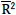=0.92
Estimation period: February 16, 2016 to July 29, 2016

As the regression coefficient of 0.60 shows, the "midpoint rate on the current day - closing rate on the preceding day" (actual value) tends to rise (fall) by only 0.6bp, when the "currency basket adjustment" (theoretical value) rises (falls) by 1bp. This implies that the weights of currencies other than the dollar in the currency basket actually used as a reference when determining the midpoint rate may only be about 60% of their corresponding weights in the CFETS RMB Index, upon which the simulation is based. In contrast, the weight of the dollar in the same basket may be much higher than its corresponding weight in the CFETS RMB Index (Hypothesis III).

To verify Hypothesis III, an adjustment is made by multiplying the weight of currencies other than the dollar in the CFETS RMB Index by 0.6 times, and then adding the reduced weight to the dollar. In this revised version of the CFETS RMB Index, the combined weight of currencies other than the dollar declines from 73.60% to 44.16%, while in contrast, the weight of the dollar increased from 26.40% to 55.84% (see Table 1). We then re-calculate the "currency basket-based adjustment" (theoretical value) based on this revised version. Because the error (absolute value) between the theoretical value so calculated and the actual value ("midpoint rate on the current day - closing rate on the preceding day") is smaller than the errors in two other hypotheses, particularly in the third period, we might say that the explanatory power of this hypothesis is stronger (Figure 5).

Figure 5: Verification of the "Closing Rate on the Preceding Day + Currency Basket-based Adjustment" Rule (Revised Version)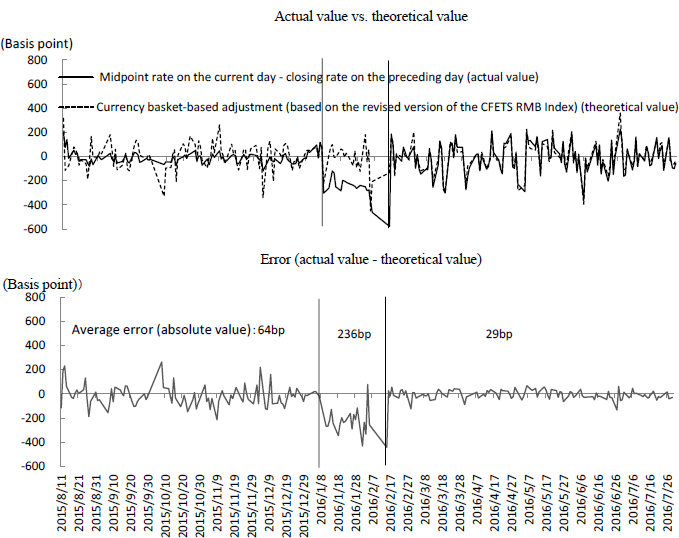Note: Weights of the currencies in the revised version of the CFETS basket are estimates made by the author.
Source: Compiled based on the CFETS (the midpoint rate on the current day, weights of the currency basket, exchange rates of currencies against the dollar) and Bloomberg (the closing rate on the preceding day).

## 4. Foreign exchange regime yet to be completed

Since 2005, China has been moving forward the foreign exchange reforms that aim to enhance the flexibility of yuan exchange rate, but the pace of these reforms has been extremely slow. As a result of implementing the "closing rate on the preceding day + currency basket-based adjustment" rule after mid-February 2016, the midpoint rate and eventually the market rate of the yuan have come to better reflect the supply-demand situation in the market and changes in the exchange rates of major currencies, making it less likely for the yuan to diverge sharply from its equilibrium rate.

Nevertheless, if the yuan faces strong upward or downward pressure from some shock, foreign exchange intervention by the authorities will be necessary to keep the market rate within the +/- 2% band around the midpoint rate. Given that this will be accompanied by changes in the foreign exchange reserves and the money supply (i.e., if the dollar is bought and the yuan is sold, the money supply will increase, and if the dollar is sold and the yuan is bought, the money supply will decrease), the independence of China's monetary policy is still constrained.

To cut off the link between foreign exchange intervention and the money supply and eventually increase the independence of the monetary policy, China must shift to a free floating exchange rate system in which the authorities stop announcing the midpoint rate and in principle do not intervene in the foreign exchange market. The implementation of the "closing rate on the preceding day + currency basket-based adjustment" rule, which has improved the flexibility of yuan exchange rate, can be viewed as a major step toward this goal.

The original text in Japanese was posted on August 15, 2016.

Footnote(s)
1. ^ For the sake of simplification, it is assumed here that the currency basket that serves as a reference consists of only two currencies: the euro and the dollar. However, if there are more currencies in the basket, changes in the exchange rates of all these currencies against the dollar must be taken into account when determining the exchange rate of the home currency against the dollar.
2. ^ Because the exchange of the dollar against the dollar always remains the same at 1 and its rate of change is zero, it can be omitted from the calculation here.
3. ^ According to the explanation made by the PBOC, the market makers estimate the midpoint rate at their own discretion. However, for the sake of simplification, no distinction has been made between the authorities and the market makers here.
4. ^ Because the exchange rate of Thai baht, a constituent currency of the CFETS, is not included, it was not taken into account when the calculation was made.
Related articles

September 30, 2016

## Article(s) by this author

• ### China Labeled as a Currency Manipulator— Is the Chinese Yuan Withering?

October 10, 2019［China in Transition］

• ### Expansion and Prolonging of U.S.-China Trade Friction—Conspicuous Trend of Decoupling

September 19, 2019［China in Transition］

• ### U.S.-China Trade Friction Casting a Shadow over the Chinese Economy—Impact on the supply side becoming a matter of concern

April 2, 2019［China in Transition］

• ### Why Has the U.S. Waged A Trade War Against China?—Protracted Confrontation Inevitable

December 4, 2018［China in Transition］

• ### Chinese Economy Slowing Down amid Intensifying U.S.-China Trade Dispute: Reform and opening-up should come before economic stimulus

October 19, 2018［China in Transition］